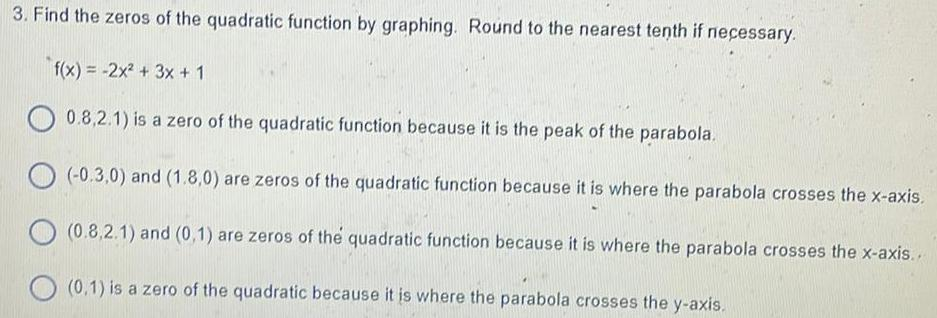Question:

# 3. Find the zeros of the quadratic function by graphing.

Last updated: 8/14/20223. Find the zeros of the quadratic function by graphing. Round to the nearest tenth if necessary. f(x) = -2x² + 3x + 1 0.8,2.1) is a zero of the quadratic function because it is the peak of the parabola. (-0.3,0) and (1.8,0) are zeros of the quadratic function because it is where the parabola crosses the x-axis. (0.8,2.1) and (0,1) are zeros of the quadratic function because it is where the parabola crosses the x-axis... (0.1) is a zero of the quadratic because it is where the parabola crosses the y-axis.